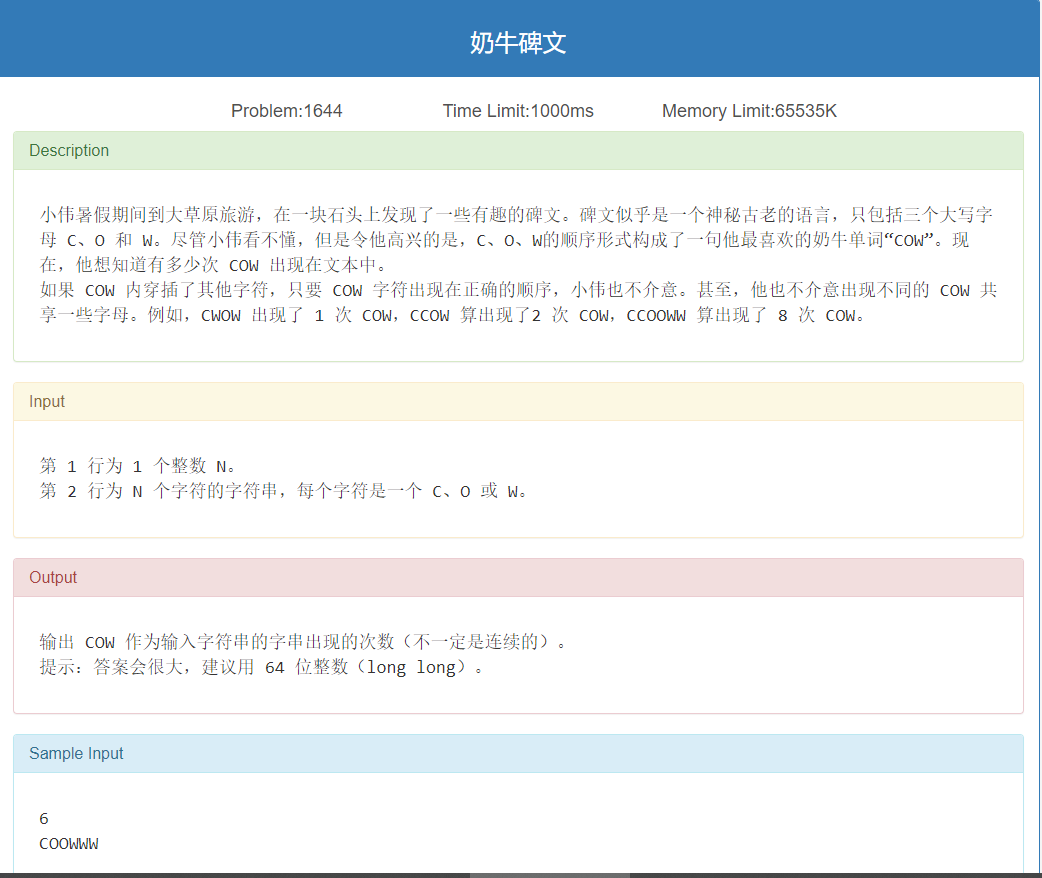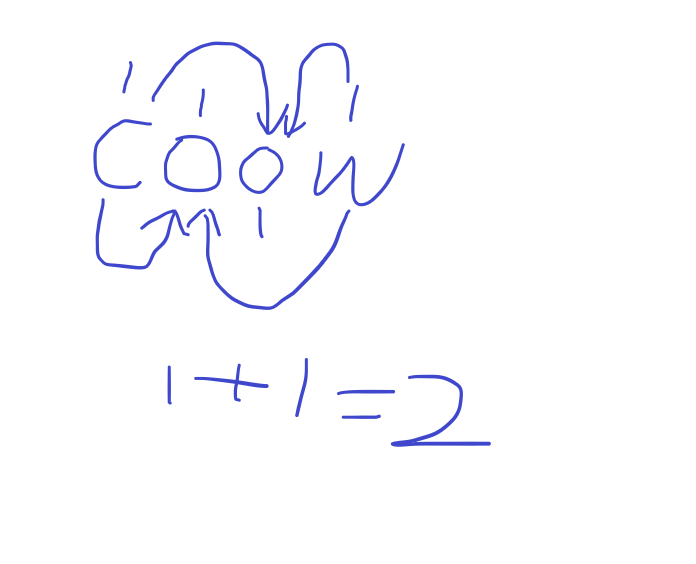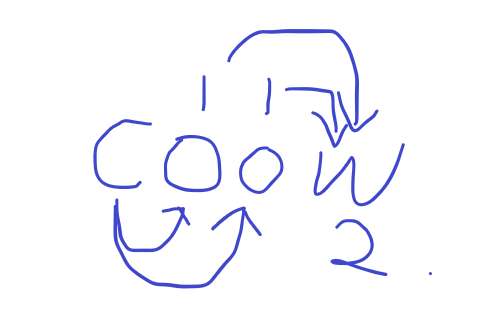# 奶牛碑文

## 奶牛碑文1. WOC: C后面没有字母,这种情况下,答案应当为0
2. WCOCOW: C出现在了O或者W的后面
3. WO: 字母不全``````#include <bits/stdc++.h>
using namespace std;

int o,w;
int main()
{
typedef long long ll;
int length;
int index_o=0,index_w=0,cnt=0,number_w=0;
char str;
cin>>length>>str;
for (int i=0;i<length;i++)
{
if (str[i]=='C') cnt++;
if (str[i]=='O') {
o[index_o]=cnt;
number_w+=cnt;
index_o++;
}
if (str[i]=='W') {
w[index_w]=number_w;
index_w++;
}
}
//cout<<cnt<<endl;
ll ans=0;
for (int i=0;i<index_w;i++){
ans+=w[i];
}
cout<<ans<<endl;
return 0;
}``````